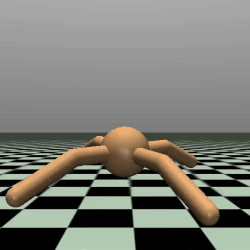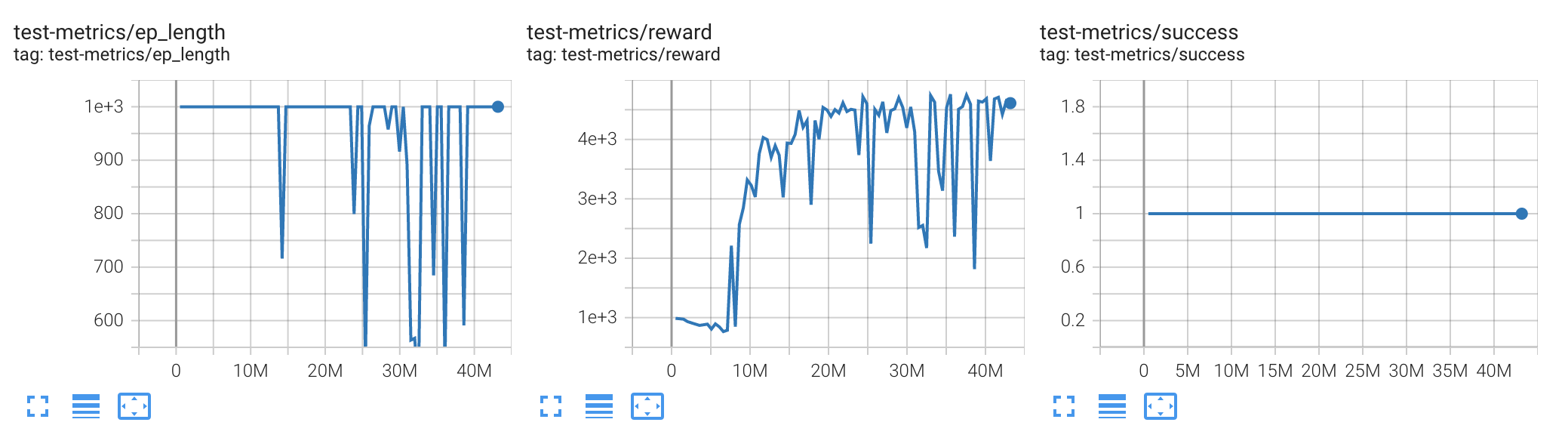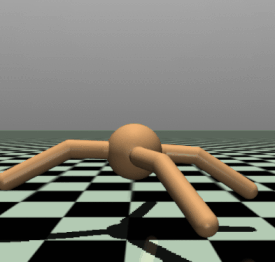Tutorial: OpenAI gym MuJoCo environment.#

Note The provided commands to execute in this tutorial assume you have installed the full library and the requirements for the gym_plugin. The latter can be installed by

pip install -r allenact_plugins/gym_plugin/extra_requirements.txt

The environments for this tutorial use MuJoCo(Multi-Joint dynamics in Contact) physics simulator, which is also required to be installed properly with instructions here.

For this tutorial, we'll focus on one of the continuous-control environments under the mujoco group of gym environments: Ant-v2. In this task, the goal is to make a four-legged creature, "ant", walk forward as fast as possible. A random agent of "Ant-v2" is shown below..

To achieve the goal, we need to provide continuous control for the agent moving forward with four legs with the x velocity as high as possible for at most 1000 episodes steps. The agent is failed, or done, if the z position is out of the range [0.2, 1.0]. The dimension of the action space is 8 and 111 for the dimension of the observation space that maps to different body parts, including 3D position (x,y,z), orientation(quaternion x,y,z,w) of the torso, and the joint angles, 3D velocity (x,y,z), 3D angular velocity (x,y,z), and joint velocities. The rewards for the agent "ant" are composed of the forward rewards, healthy rewards, control cost, and contact cost.

Implementation#

For this tutorial, we'll use the readily available gym_plugin, which includes a wrapper for gym environments, a task sampler and task definition, a sensor to wrap the observations provided by the gym environment, and a simple model. The experiment config, similar to the one used for the Navigation in MiniGrid tutorial, is defined as follows:

from typing import Dict, Optional, List, Any, cast

import gym
import torch.nn as nn
import torch.optim as optim
from torch.optim.lr_scheduler import LambdaLR

from allenact.algorithms.onpolicy_sync.losses.ppo import PPO

from allenact.base_abstractions.sensor import SensorSuite
from allenact_plugins.gym_plugin.gym_models import MemorylessActorCritic
from allenact_plugins.gym_plugin.gym_sensors import GymMuJoCoSensor

from allenact.utils.experiment_utils import (
TrainingPipeline,
Builder,
PipelineStage,
LinearDecay,
)
from allenact.utils.viz_utils import VizSuite, AgentViewViz

class HandManipulateTutorialExperimentConfig(ExperimentConfig):
@classmethod
def tag(cls) -> str:
return "GymMuJoCoTutorial"

Sensors and Model#

As mentioned above, we'll use a GymBox2DSensor to provide full observations from the state of the gym environment to our model.

SENSORS = [
GymMuJoCoSensor("Ant-v2", uuid="gym_mujoco_data"),
]

We define our ActorCriticModel agent using a lightweight implementation with separate MLPs for actors and critic, MemorylessActorCritic. Since this is a model for continuous control, note that the superclass of our model is ActorCriticModel[GaussianDistr] instead of ActorCriticModel[CategoricalDistr], since we'll use a Gaussian distribution to sample actions.

@classmethod
def create_model(cls, **kwargs) -> nn.Module:
"""We define our `ActorCriticModel` agent using a lightweight
implementation with separate MLPs for actors and critic,
MemorylessActorCritic.

Since this is a model for continuous control, note that the
superclass of our model is `ActorCriticModel[GaussianDistr]`
instead of `ActorCriticModel[CategoricalDistr]`, since we'll use
a Gaussian distribution to sample actions.
"""
return MemorylessActorCritic(
input_uuid="gym_mujoco_data",
action_space=gym.spaces.Box(
-3.0, 3.0, (8,), "float32"
),  # 8 actors, each in the range [-3.0, 3.0]
observation_space=SensorSuite(cls.SENSORS).observation_spaces,
action_std=0.5,
)

We use an available TaskSampler implementation for gym environments that allows to sample GymTasks: GymTaskSampler. Even though it is possible to let the task sampler instantiate the proper sensor for the chosen task name (by passing None), we use the sensors we created above, which contain a custom identifier for the actual observation space (gym_mujoco_data) also used by the model.

@classmethod

For convenience, we will use a _get_sampler_args method to generate the task sampler arguments for all three modes, train, valid, test:

self,
process_ind: int,
total_processes: int,
devices: Optional[List[int]] = None,
seeds: Optional[List[int]] = None,
deterministic_cudnn: bool = False,
) -> Dict[str, Any]:
return self._get_sampler_args(
process_ind=process_ind, mode="train", seeds=seeds
)

self,
process_ind: int,
total_processes: int,
devices: Optional[List[int]] = None,
seeds: Optional[List[int]] = None,
deterministic_cudnn: bool = False,
) -> Dict[str, Any]:
return self._get_sampler_args(
process_ind=process_ind, mode="valid", seeds=seeds
)

self,
process_ind: int,
total_processes: int,
devices: Optional[List[int]] = None,
seeds: Optional[List[int]] = None,
deterministic_cudnn: bool = False,
) -> Dict[str, Any]:
return self._get_sampler_args(process_ind=process_ind, mode="test", seeds=seeds)

Similarly to what we do in the Minigrid navigation tutorial, the task sampler samples random tasks for ever, while, during testing (or validation), we sample a fixed number of tasks.

def _get_sampler_args(
self, process_ind: int, mode: str, seeds: List[int]
) -> Dict[str, Any]:
"""Generate initialization arguments for train, valid, and test

# Parameters
process_ind : index of the current task sampler
mode:  one of `train`, `valid`, or `test`
"""
if mode == "train":
task_seeds_list = None  # no predefined random seeds for training
deterministic_sampling = False  # randomly sample tasks in training
else:

# one seed for each task to sample:
# - ensures different seeds for each sampler, and
# - ensures a deterministic set of sampled tasks.
)

deterministic_sampling = (
True  # deterministically sample task in validation/testing
)

return dict(
gym_env_types=["Ant-v2"],
sensors=self.SENSORS,  # sensors used to return observations to the agent
deterministic_sampling=deterministic_sampling,  # see above
seed=seeds[process_ind],
)

Note that we just sample 4 tasks for validation and testing in this case, which suffice to illustrate the model's success.

Machine parameters#

In this tutorial, we just train the model on the CPU. We allocate a larger number of samplers for training (8) than for validation or testing (just 1), and we default to CPU usage by returning an empty list of devices. We also include a video visualizer (AgentViewViz) in test mode.

@classmethod
def machine_params(cls, mode="train", **kwargs) -> Dict[str, Any]:
visualizer = None
if mode == "test":
visualizer = VizSuite(
mode=mode,
video_viz=AgentViewViz(
label="episode_vid",
max_clip_length=400,
fps=30,
),
)
return {
"nprocesses": 8 if mode == "train" else 1,  # rollout
"devices": [],
"visualizer": visualizer,
}

Training pipeline#

The last definition is the training pipeline. In this case, we use a PPO stage with linearly decaying learning rate and 10 single-batch update repeats per rollout. The reward should exceed 4,000 in 20M steps in the test. In order to make the "ant" run with an obvious fast speed, we train the agents using PPO with 3e7 steps.

@classmethod
def training_pipeline(cls, **kwargs) -> TrainingPipeline:
lr = 3e-4
ppo_steps = int(3e7)
clip_param = 0.2
value_loss_coef = 0.5
entropy_coef = 0.0
num_mini_batch = 4  # optimal 64
update_repeats = 10
num_steps = 2048
gamma = 0.99
use_gae = True
gae_lambda = 0.95
save_interval = 200000
metric_accumulate_interval = 50000
return TrainingPipeline(
named_losses=dict(
ppo_loss=PPO(
clip_param=clip_param,
value_loss_coef=value_loss_coef,
entropy_coef=entropy_coef,
),
),  # type:ignore
pipeline_stages=[
PipelineStage(loss_names=["ppo_loss"], max_stage_steps=ppo_steps),
],
num_mini_batch=num_mini_batch,
update_repeats=update_repeats,
num_steps=num_steps,
gamma=gamma,
use_gae=use_gae,
gae_lambda=gae_lambda,
save_interval=save_interval,
metric_accumulate_interval=metric_accumulate_interval,
lr_scheduler_builder=Builder(
LambdaLR, {"lr_lambda": LinearDecay(steps=ppo_steps, startp=1, endp=0)},
),
)

Training and validation#

We have a complete implementation of this experiment's configuration class in projects/tutorials/gym_mujoco_tutorial.py. To start training from scratch, we just need to invoke

PYTHONPATH=. python allenact/main.py gym_mujoco_tutorial -b projects/tutorials -m 8 -o /PATH/TO/gym_mujoco_output -s 0 -e

from the allenact root directory. Note that we include -e to enforce deterministic evaluation. Please refer to the Navigation in MiniGrid tutorial if in doubt of the meaning of the rest of parameters.

If we have Tensorboard installed, we can track progress with

tensorboard --logdir /PATH/TO/gym_mujoco_output

which will default to the URL http://localhost:6006/.

After 30,000,000 steps, the script will terminate. If everything went well, the valid success rate should be 1 and the mean reward to above 4,000 in 20,000,000 steps, while the average episode length should stay or a little below 1,000.

Testing#

The training start date for the experiment, in YYYY-MM-DD_HH-MM-SS format, is used as the name of one of the subfolders in the path to the checkpoints, saved under the output folder. In order to evaluate (i.e. test) a collection of checkpoints, we need to pass the --eval flag and specify the directory containing the checkpoints with the --checkpoint CHECKPOINT_DIR option:

PYTHONPATH=. python allenact/main.py gym_mujoco_tutorial \
-b projects/tutorials \
-m 1 \
-o /PATH/TO/gym_mujoco_output \
-s 0 \
-e \
--eval \
--checkpoint /PATH/TO/gym_mujoco_output/checkpoints/GymMuJoCoTutorial/YOUR_START_DATE

If everything went well, the test success rate should converge to 1, the test success rate should be 1 and the mean reward to above 4,000 in 20,000,000 steps, while the average episode length should stay or a little below 1,000. The gif results can be seen in the image tab of Tensorboard while testing. The output should be something like this:.

And the gif results can be seen in the image tab of Tensorboard while testing.If the test command fails with pyglet.canvas.xlib.NoSuchDisplayException: Cannot connect to "None", e.g. when running remotely, try prepending DISPLAY=:0.0 to the command above, assuming you have an xserver running with such display available:

DISPLAY=:0.0 PYTHONPATH=. python allenact/main.py gym_mujoco_tutorial \
-b projects/tutorials \
-m 1 \
-o /PATH/TO/gym_mujoco_output \
-s 0 \
-e \
--eval \
--checkpoint /PATH/TO/gym_mujoco_output/checkpoints/GymMuJoCoTutorial/YOUR_START_DATE